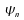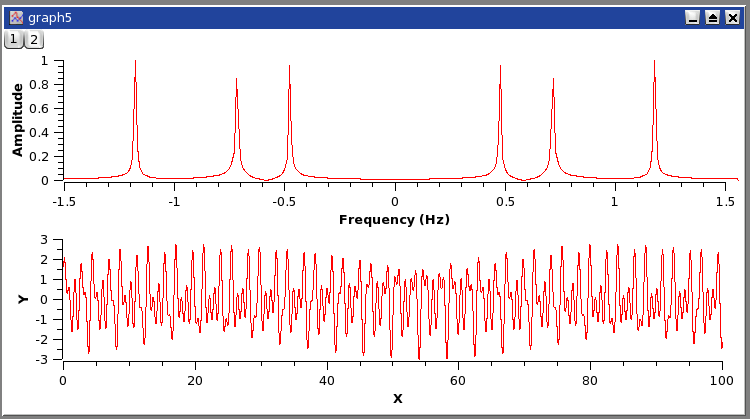Chapter 6. Analysis of data and curves

Fast Fourier Transform
Correlation
Convolution
Deconvolution
Data Fitting
Fitting to specific curves
Multi-Peaks fitting
Filtering of data curves
Interpolation

Fast Fourier Transform

This function is accessed with the FFT... command, which is located in the Analysis Menu whenever a table or a plot is selected. The Fourier transform decomposes a signal into its elementary components by assuming that the signal x(t) can be described as a sum:

Equation 6-1. Fourier equationin whichare the frequencies, an are the amplitudes of each frequency andare the phases of each frequency. QtiPlot will compute these parameters and build a new plot of the amplitude as a function of frequency.

Figure 6-1. An example of the FFT.

This FFT was performed on a curve to extract it's characteristic frequency components. The original signal is on the bottom plot, while the amplitude-frequency plot is on the top layer. In this example, the amplitude curve has been normalized, and the frequencies have been shifted to obtain a centered x-scale.The important parameters of the FFT can be modified using the FFT dialog, including the selection of an inverse FFT. An inverse FFT performed on the results of a forward FFT should result in the original signal. Frequently, it is useful to remove or modify some of the frequency components before the inverse FFT is performed. This may be particularly useful when it is desirable to remove some known interference. A common example would be the removal of power-line interference (usually 50 or 60 Hz, depending upon where you live). You should remember that this will also remove any real portions of the signal that happen to be at that same frequency. Care should be used when doing this.